Chapter 4 Part 2 - Income Determination and Multiplier

Economics Class 12
Macroeconomics

We know that

At equilibrium level

In this case,

Savings are also equal to Investment

S = I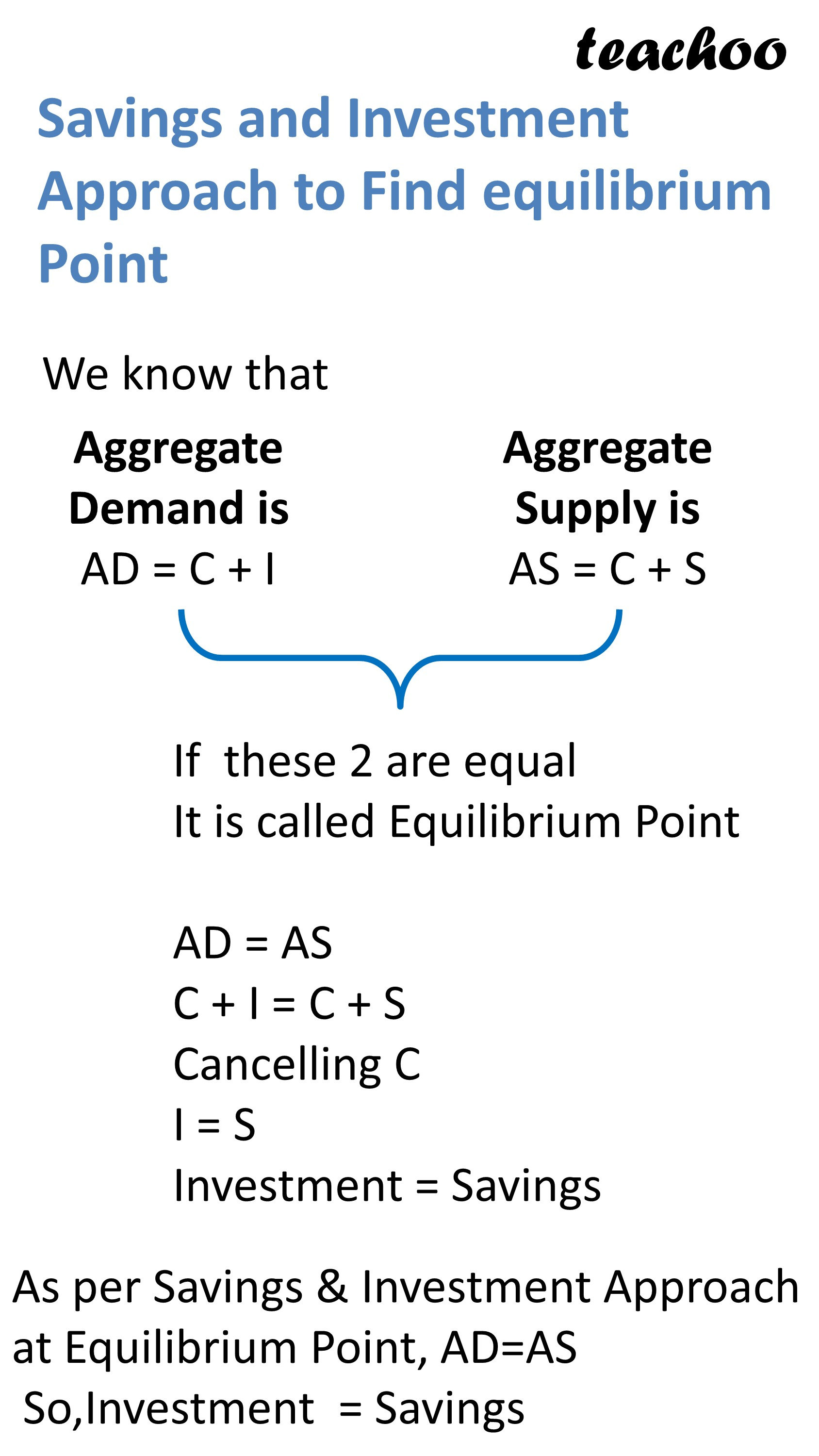## Reason

Aggregate Demand = Consumption Expenditure + Investment Expenditure

Aggregate Supply = Consumption + Saving

AS = C + S

Now, we know that

At equilibrium level

hence, we can write

C + I = C + S

Cancelling C

I = S

Investment = Savings

Lets learn how to make Schedule using this approach

## How to Make Schedule Graph of Savings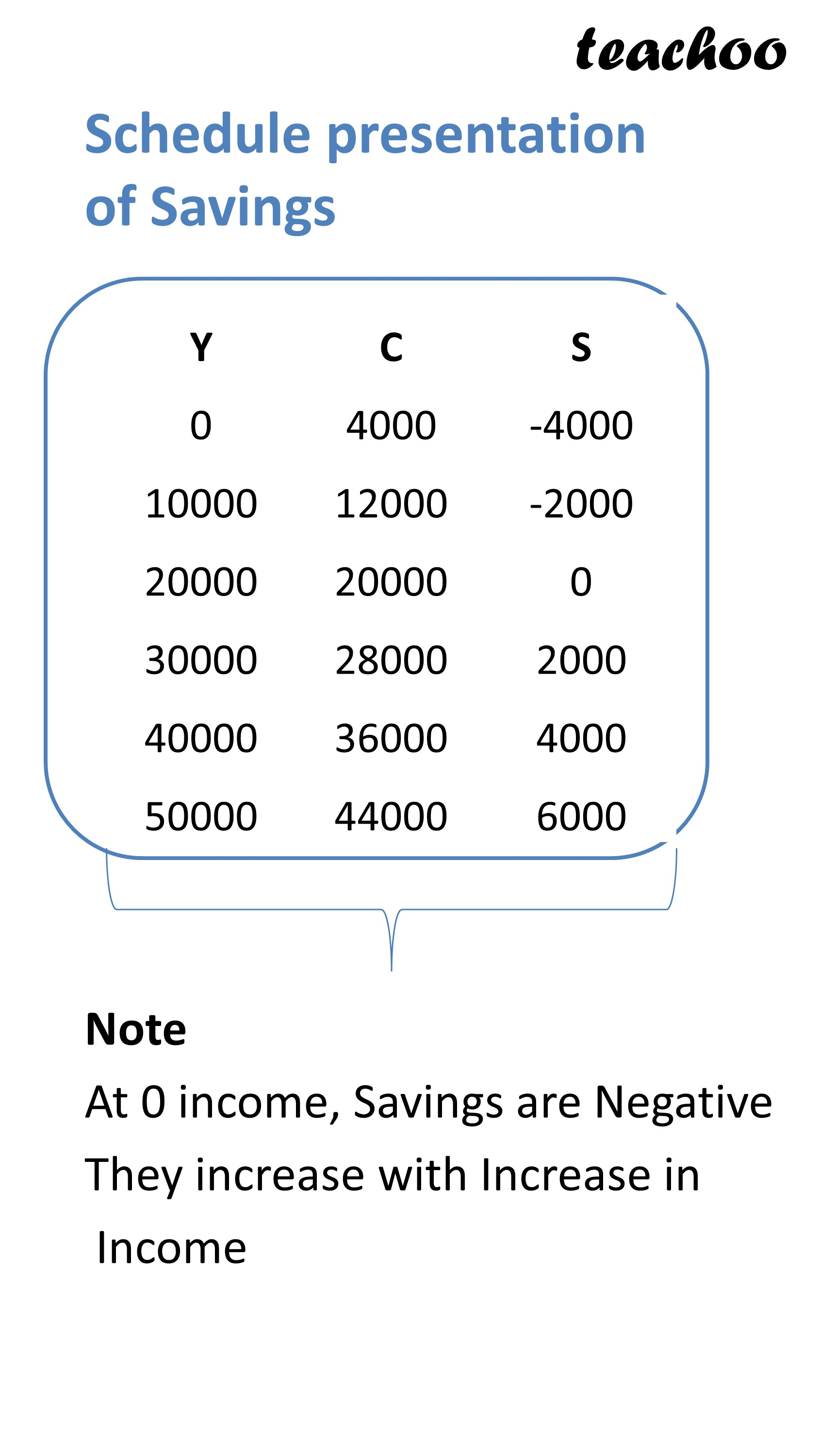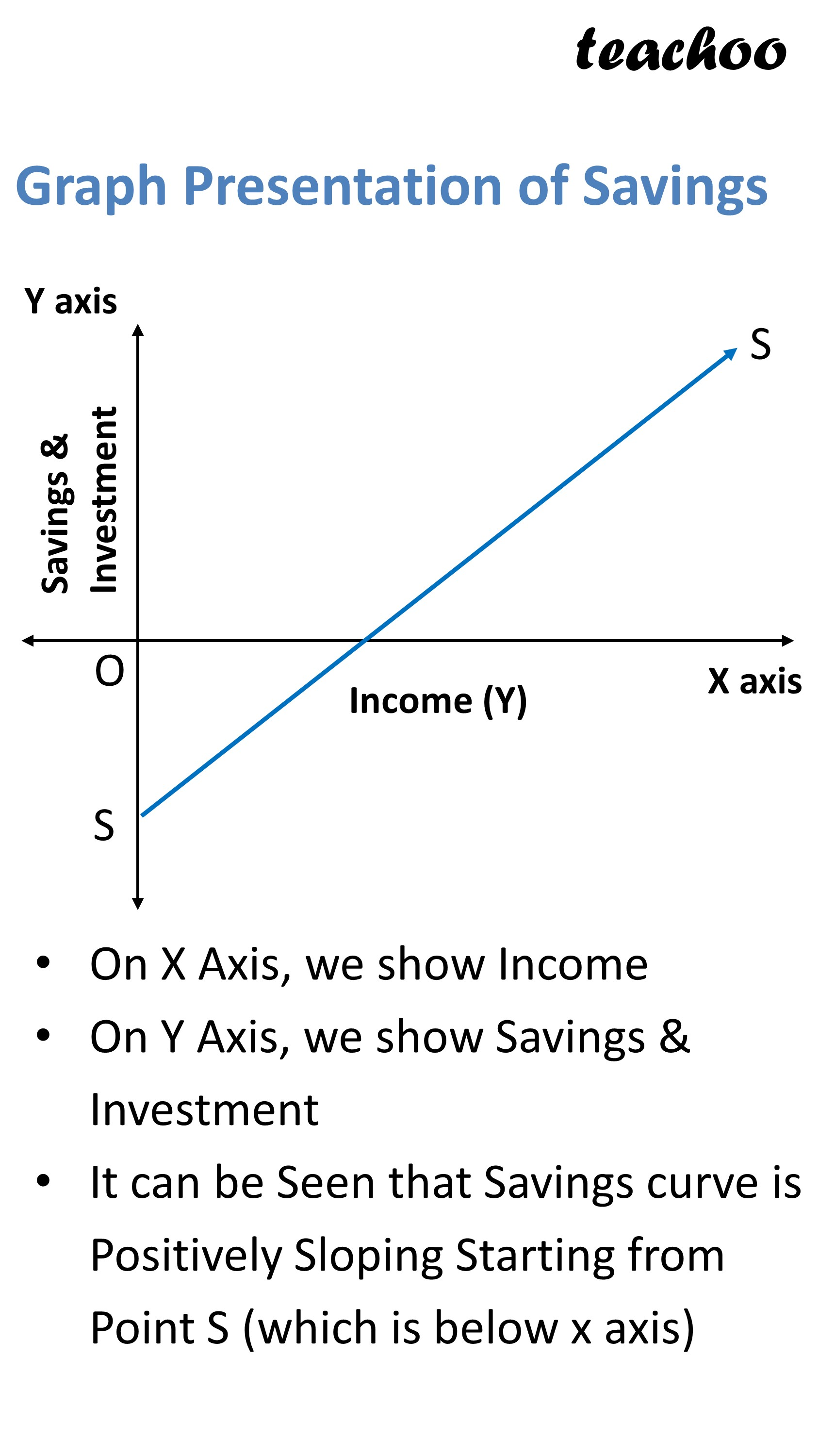## How to Make Schedule Graph of investment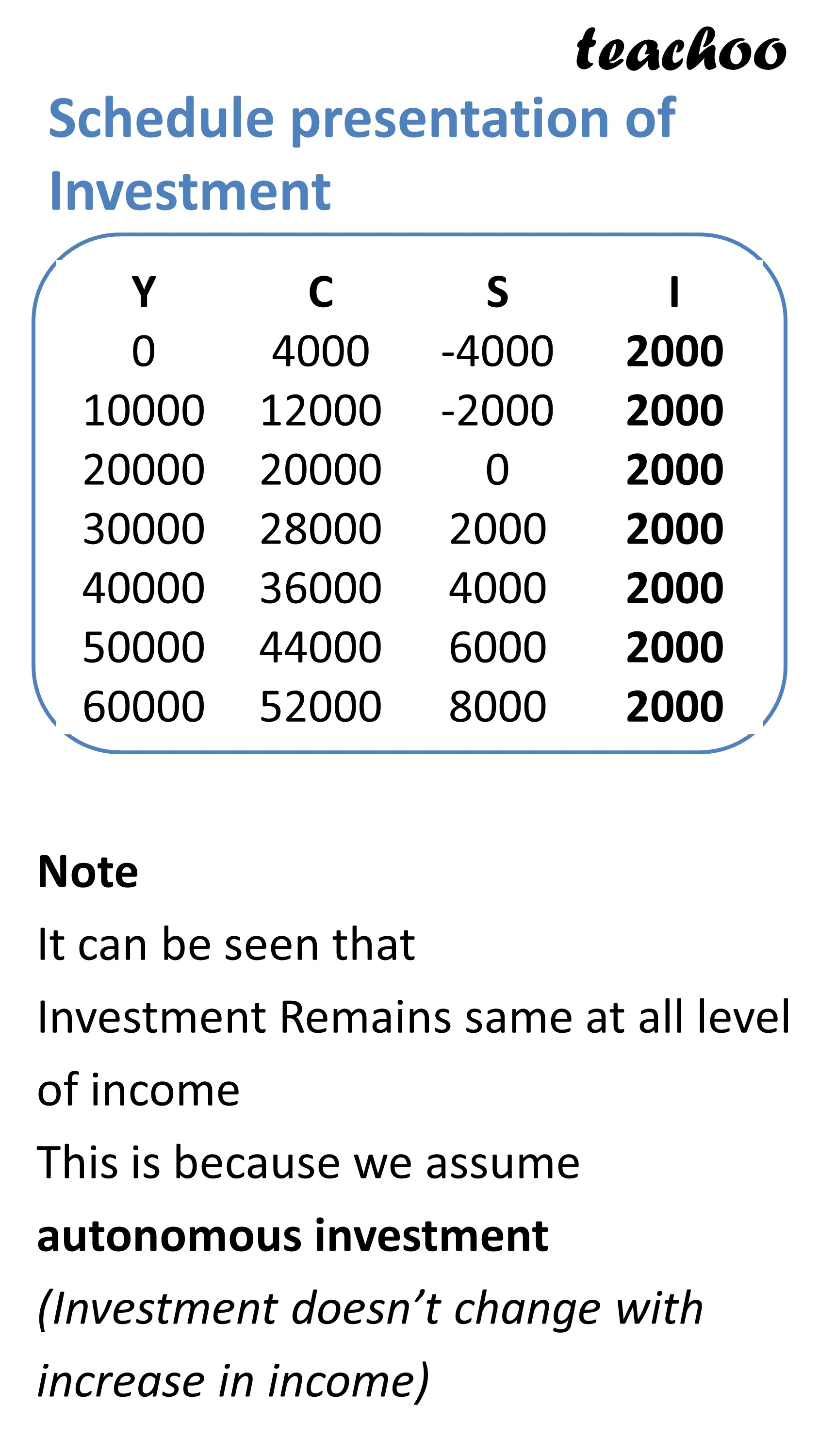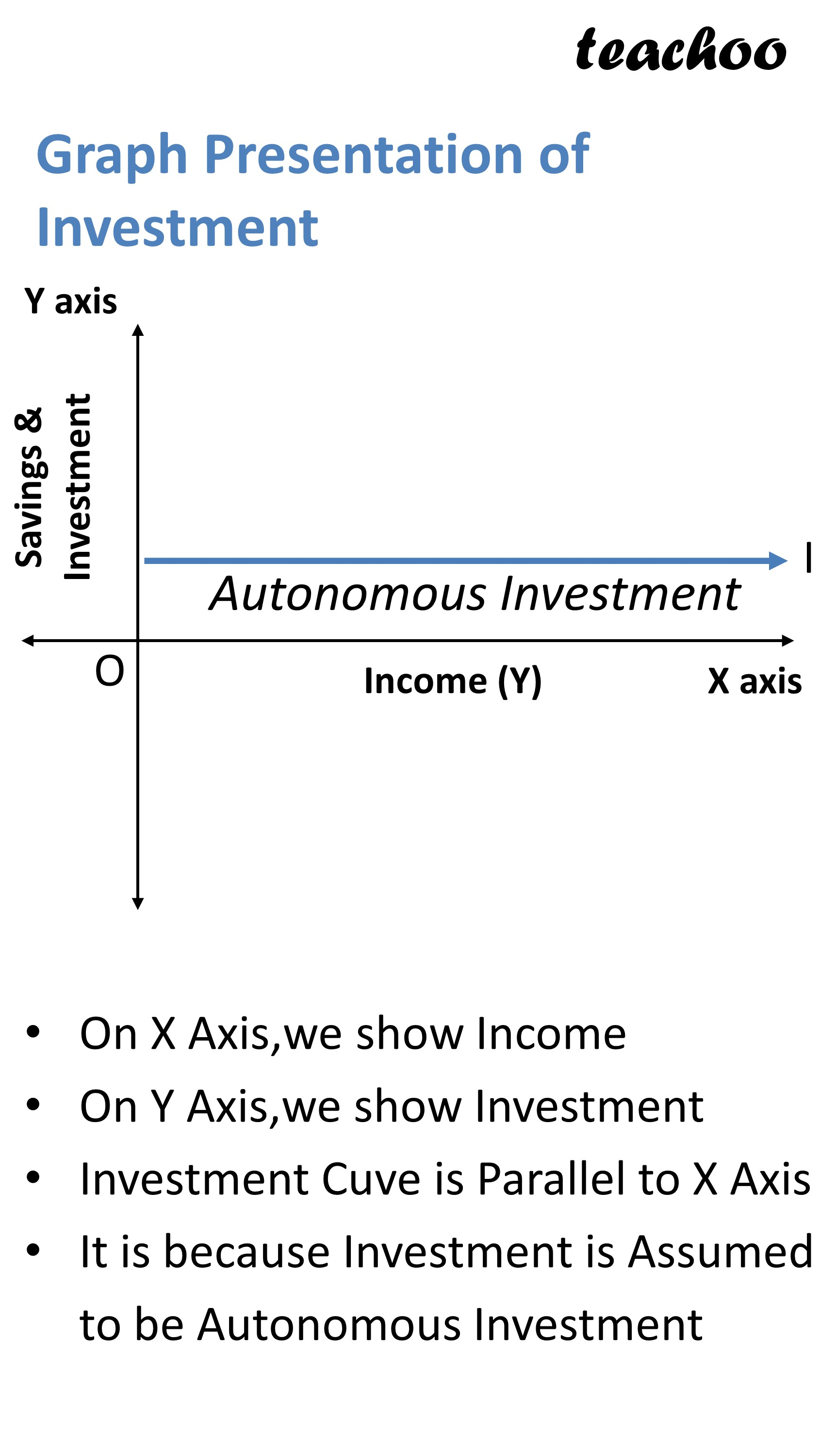Now lets make both Savings and Investment in Same graph

## Graph of Savings and Investment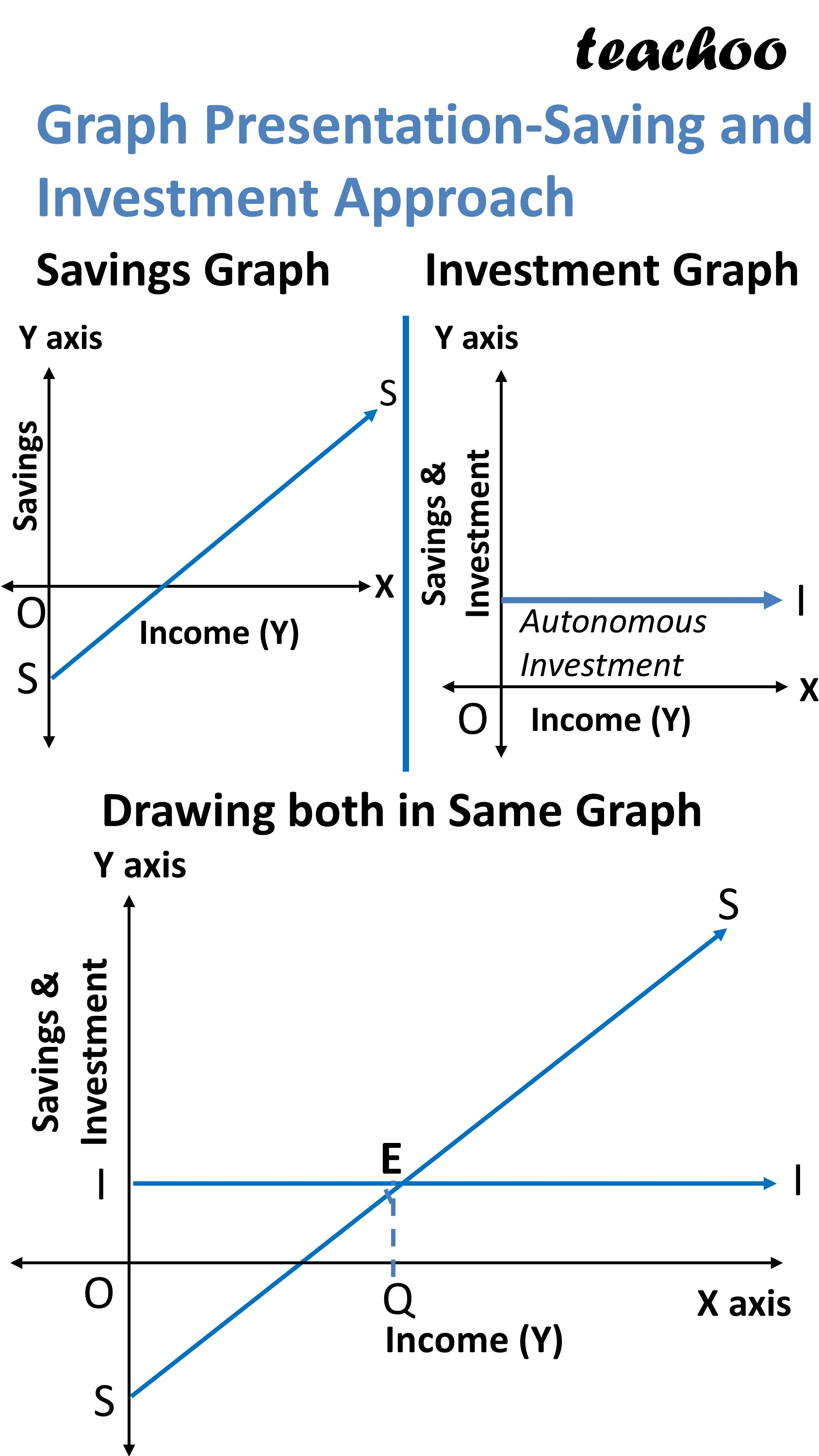On X Axis, we show Income

On Y Axis, we show Savings and Investment

It can be Seen that

Savings curve is Positively Sloping Starting from Point Y

It starts below the origin as at level of income, Savings are negative

Investment Curve is Parallel to X Axis

It is because Investment is Assumed to be Autonomous Investment

Investment Amount does not change with increase in Income

Point where Savings and Investment Curve intersect is called Equilibrium Point

We Say, at equilibrium Point, Savings=investment

At this Point, we draw a line EY connecting x axis at Point Y

This Y is equilibrium Level of income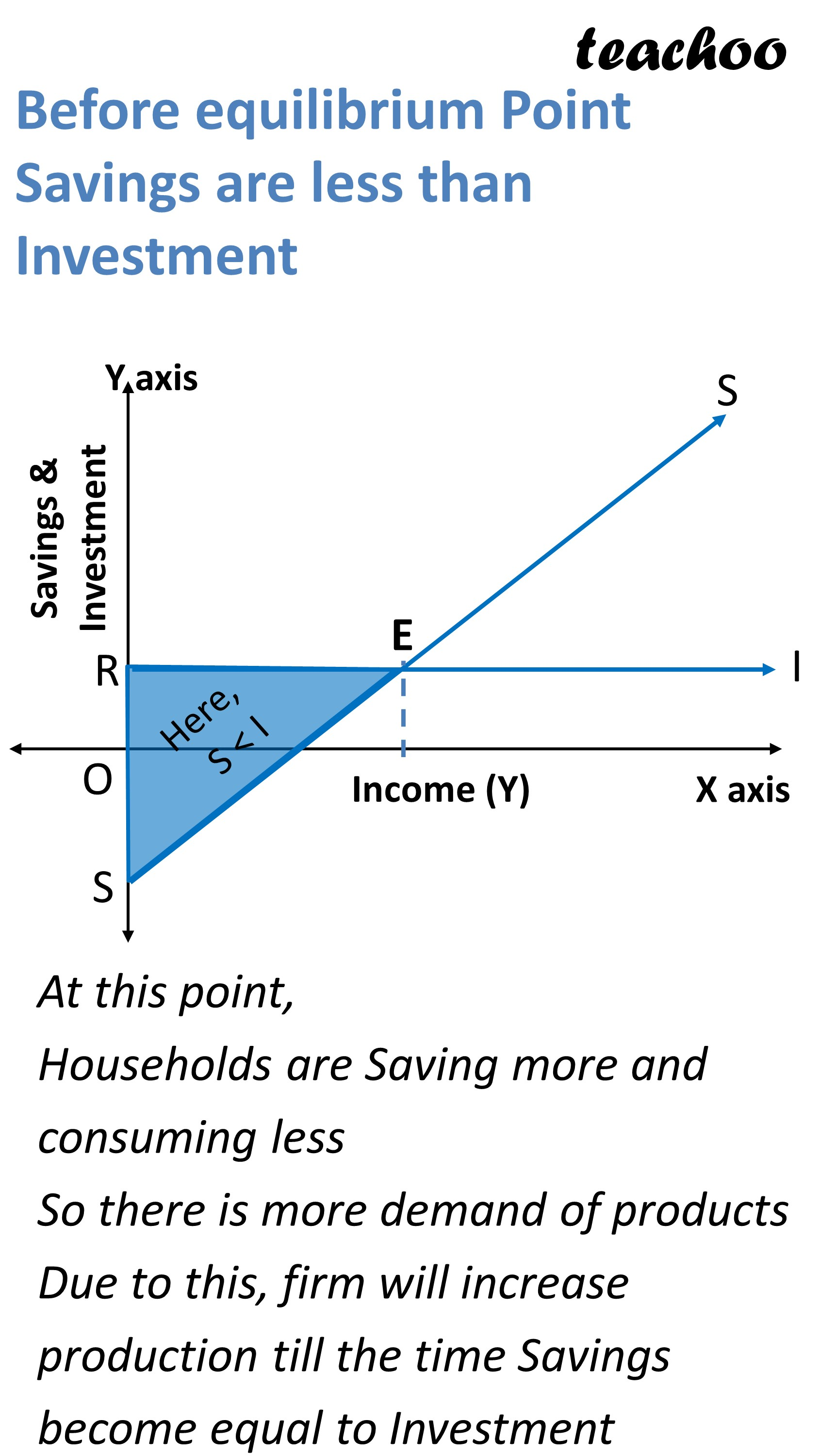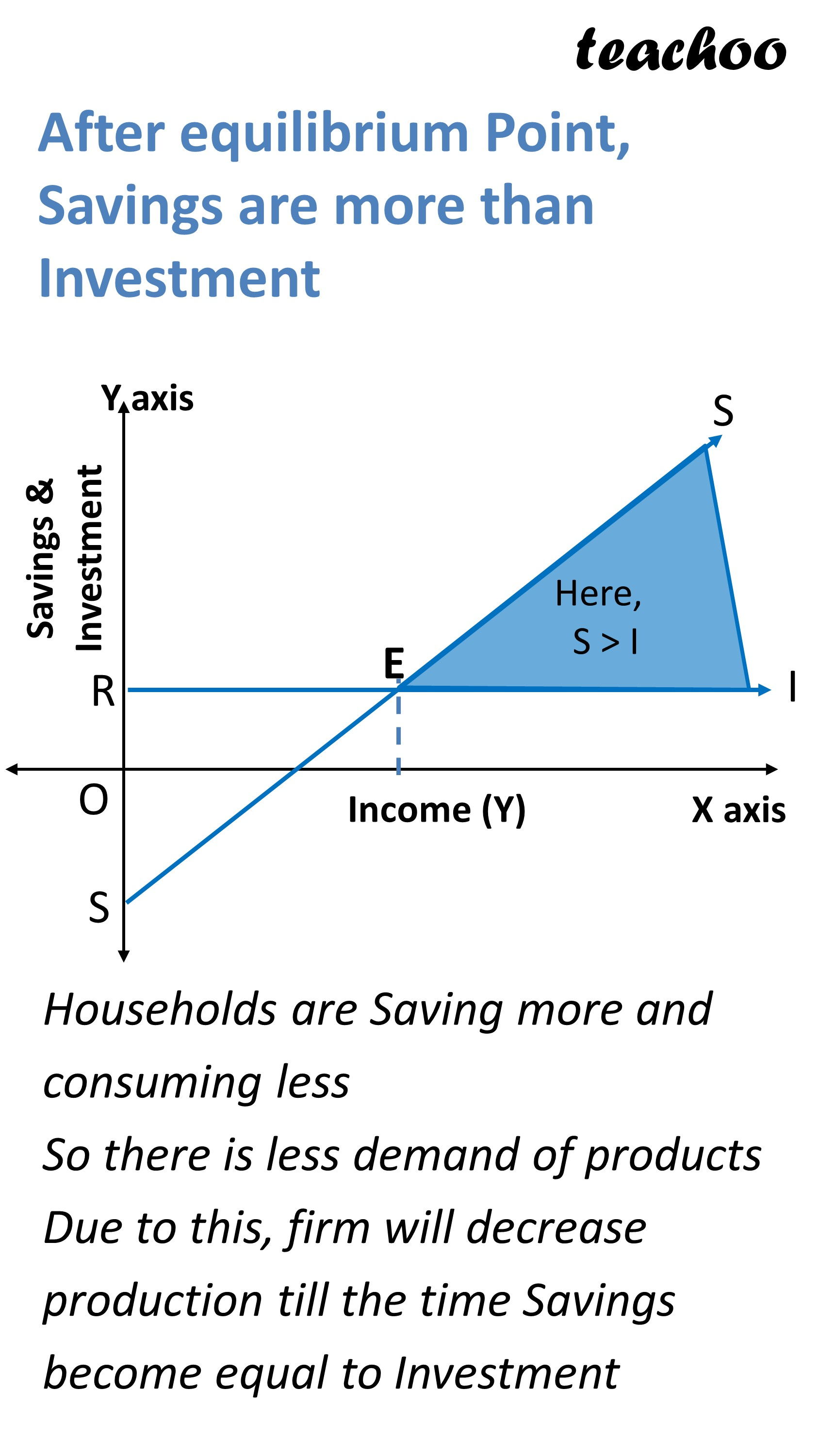### NCERT Questions

No questions in this part\

### Other Books

#### Question 1

How is equilibrium level of income attained through savings and investment approach?

Learn in your speed, with individual attention - Teachoo Maths 1-on-1 Class

### Transcript

Savings and Investment Approach to Find equilibrium Point We know that Aggregate Demand is AD = C + I Aggregate Supply is AS = C + S If these 2 are equal It is called Equilibrium Point AD = AS C + I = C + S Cancelling C I = S Investment = Savings As per Savings & Investment Approach at Equilibrium Point, AD=AS So, Investment = Savings Equilibrium Point-Schedule-As per Savings and investment Approach What is Equilibrium point in following schedule? Equilibrium Point is calculated at Point where Investment = Savings It is at income level of 30000 Schedule presentation of Savings Note At 0 income, Savings are Negative They increase with Increase in Income Graph Presentation of Savings On X Axis, we show Income On Y Axis, we show Savings & Investment It can be Seen that Savings curve is Positively Sloping Starting from Point S (which is below x axis) Schedule presentation of Investment Note It can be seen that Investment Remains same at all level of income This is because we assume autonomous investment (Investment doesn’t change with increase in income) Graph Presentation of Investment Autonomous Investment On X Axis, we show Income On Y Axis, we show Investment Investment Curve is Parallel to X Axis It is because Investment is Assumed to be Autonomous Investment Graph Presentation-Saving and Investment Approach Savings Graph Investment Graph Drawing both in Same Graph Before equilibrium Point Savings are less than Investment At this point, Households are Saving more and consuming less So there is more demand of products Due to this, firm will increase production till the time Savings become equal to Investment Savings & Investment After equilibrium Point, Savings are more than Investment Households are Saving more and consuming less So there is less demand of products Due to this, firm will decrease production till the time Savings become equal to Investment Comparison of AD-AS Graph and Savings Investment Graph